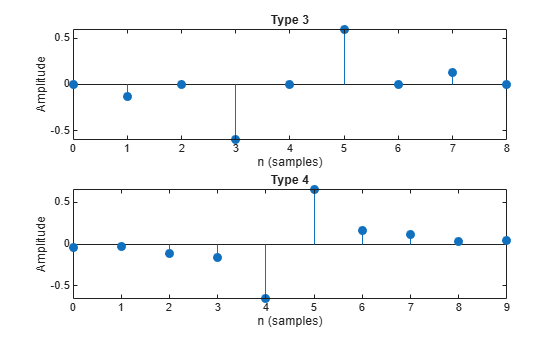# firtype

Type of linear phase FIR filter

## Syntax

``t = firtype(b)``
``t = firtype(d)``

## Description

example

````t = firtype(b)` determines the type, `t`, of an FIR filter with coefficients `b`. `t` can be 1, 2, 3, or 4. The filter must be real and have linear phase.```

example

````t = firtype(d)` determines the type, `t`, of an FIR filter, `d`. `t` can be 1, 2, 3, or 4. The filter must be real and have linear phase.```

## Examples

collapse all

Design two FIR filters using the window method, one of even order and the other of odd order. Determine their types and plot their impulse responses.

```subplot(2,1,1) b = fir1(8,0.5); impz(b), title(['Type ' int2str(firtype(b))]) subplot(2,1,2) b = fir1(9,0.5); impz(b), title(['Type ' int2str(firtype(b))])```Design two equiripple Hilbert transformers, one of even order and the other of odd order. Determine their types and plot their impulse responses.

```subplot(2,1,1) b = firpm(8,[0.2 0.8],[1 1],'hilbert'); impz(b), title(['Type ' int2str(firtype(b))]) subplot(2,1,2) b = firpm(9,[0.2 0.8],[1 1],'hilbert'); impz(b), title(['Type ' int2str(firtype(b))])```Use `designfilt` to design the filters from the previous example. Display their types.

```d1 = designfilt('lowpassfir','DesignMethod','window', ... 'FilterOrder',8,'CutoffFrequency',0.5); disp(['d1 is of type ' int2str(firtype(d1))])```
```d1 is of type 1 ```
```d2 = designfilt('lowpassfir','DesignMethod','window', ... 'FilterOrder',9,'CutoffFrequency',0.5); disp(['d2 is of type ' int2str(firtype(d2))])```
```d2 is of type 2 ```
```d3 = designfilt('hilbertfir','DesignMethod','equiripple', ... 'FilterOrder',8,'TransitionWidth',0.4); disp(['d3 is of type ' int2str(firtype(d3))])```
```d3 is of type 3 ```
```d4 = designfilt('hilbertfir','DesignMethod','equiripple', ... 'FilterOrder',9,'TransitionWidth',0.4); disp(['d4 is of type ' int2str(firtype(d4))])```
```d4 is of type 4 ```

## Input Arguments

collapse all

Filter coefficients of the FIR filter, specified as a double- or single-precision real-valued row or column vector.

Data Types: `double` | `single`

FIR filter, specified as a `digitalFilter` object. Use `designfilt` to generate a digital filter based on frequency-response specifications.

## Output Arguments

collapse all

Filter type, returned as either 1, 2, 3, or 4. Filter types are defined as follows:

• Type 1 — Even-order symmetric coefficients

• Type 2 — Odd-order symmetric coefficients

• Type 3 — Even-order antisymmetric coefficients

• Type 4 — Odd-order antisymmetric coefficients